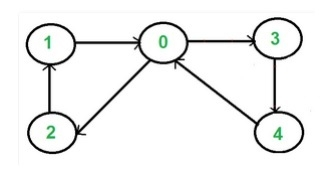C++ Program to Check Whether a Directed Graph Contains a Eulerian Cycle

C++Server Side ProgrammingProgramming

The Euler cycle/circuit is a path; by which we can visit every edge exactly once. We can use the same vertices for multiple times. The Euler Circuit is a special type of Euler path. When the starting vertex of the Euler path is also connected with the ending vertex of that path, then it is called the Euler Circuit.To check whether a graph is Eulerian or not, we have to check two conditions −

• The graph must be connected.

• The in-degree and out-degree of each vertex must be same.

Input − Adjacency matrix of the graph.

 0 1 0 0 0 0 0 1 0 0 0 0 0 1 1 1 0 0 0 0 0 0 1 0 0

Output − Euler Circuit Found

Algorithm

traverse(u, visited)

Input − The start node u and the visited node to mark which node is visited.

Output − Traverse all connected vertices.

Begin
mark u as visited
for all vertex v, if it is adjacent with u, do
if v is not visited, then
traverse(v, visited)
done
End

isConnected(graph)

Input − The graph.

Output − True if the graph is connected.

Begin
define visited array
for all vertices u in the graph, do
make all nodes unvisited
traverse(u, visited)
if any unvisited node is still remaining, then
return false
done
return true
End

isEulerCircuit(Graph)

Input − The given Graph.

Output − True when one Euler circuit is found.

Begin
if isConnected() is false, then
return false
define list for inward and outward edge count for each node
for all vertex i in the graph, do
sum := 0
for all vertex j which are connected with i, do
inward edges for vertex i increased
increase sum
done
number of outward of vertex i is sum
done
if inward list and outward list are same, then
return true
otherwise return false
End

Example Code

#include<iostream>
#include<vector>
#define NODE 5
using namespace std;
int graph[NODE][NODE] = {{0, 1, 0, 0, 0},
{0, 0, 1, 0, 0},
{0, 0, 0, 1, 1},
{1, 0, 0, 0, 0},
{0, 0, 1, 0, 0}};
void traverse(int u, bool visited[]) {
visited[u] = true;     //mark v as visited
for(int v = 0; v<NODE; v++) {
if(graph[u][v]) {
if(!visited[v])
traverse(v, visited);
}
}
}
bool isConnected() {
bool *vis = new bool[NODE];
//for all vertex u as start point, check whether all nodes are visible or not
for(int u; u < NODE; u++) {
for(int i = 0; i<NODE; i++)
vis[i] = false;     //initialize as no node is visited
traverse(u, vis);
for(int i = 0; i<NODE; i++) {
if(!vis[i])     //if there is a node, not visited by traversal, graph is not connected
return false;
}
}
return true;
}
bool isEulerCircuit() {
if(isConnected() == false) {     //when graph is not connected
return false;
}
vector<int> inward(NODE, 0), outward(NODE, 0);
for(int i = 0; i<NODE; i++) {
int sum = 0;
for(int j = 0; j<NODE; j++) {
if(graph[i][j]) {
inward[j]++;     //increase inward edge for destination
vertex
sum++;    //how many outward edge
}
}
outward[i] = sum;
}
if(inward == outward)      //when number inward edges and outward edges
for each node is same
return true;
return false;
}
int main() {
if(isEulerCircuit())
cout << "Euler Circuit Found.";
else
cout << "There is no Euler Circuit.";
}

Output

Euler Circuit Found.